Courses

# The Force Method of Analysis: Beams - 2 Civil Engineering (CE) Notes | EduRev

## Civil Engineering (CE) : The Force Method of Analysis: Beams - 2 Civil Engineering (CE) Notes | EduRev

The document The Force Method of Analysis: Beams - 2 Civil Engineering (CE) Notes | EduRev is a part of the Civil Engineering (CE) Course Structural Analysis.
All you need of Civil Engineering (CE) at this link: Civil Engineering (CE)

Example 8.1

Calculate the support reactions in the continuous beam ABC due to loading as shown in Fig. 8.2a. Assume EI to be constant throughout.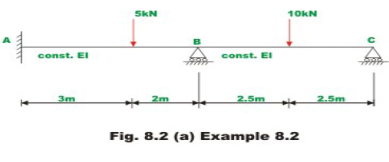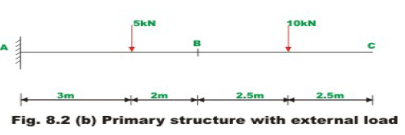Select two reactions viz, at B(R1) and C(R2) as redundants, since the given beam is statically indeterminate to second degree. In this case the primary structure is a cantilever beam AC. The primary structure with a given loading is shown in Fig. 8.2b.

In the present case, the deflections (ΔL)1, and (ΔL)2  of the released structure at B and C can be readily calculated by moment-area method. Thus,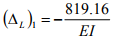and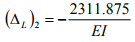For the present problem the flexibility matrix is,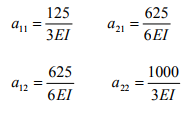(2)

In the actual problem the displacements at B and C are zero. Thus the compatibility conditions for the problem may be written as,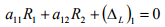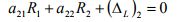(3)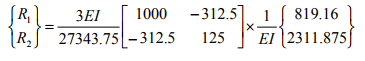(5)

Substituting the value of E and I in the above equation,

R1 =10.609kN and R2 = 3.620 kN

Using equations of static equilibrium,

R3 = 0.771 kN and R4 = −0.755 kN.m

Example 8.2

A clamped beam AB of constant flexural rigidity is shown in Fig. 8.3a. The beam is subjected to a uniform distributed load of w kN/m and a central concentrated moment M = wL2 kN.m Draw shear force and bending moment diagrams by force method.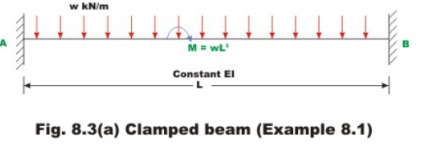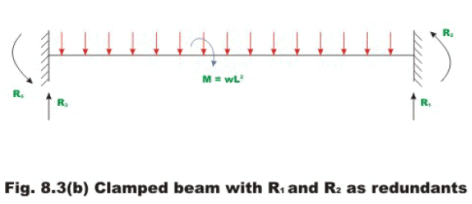Select vertical reaction (R1) and the support moment (R2) at B as the redundants. The primary structure in this case is a cantilever beam which could be obtained by releasing the redundants R1 and R2. The R1 is assumed to be positive in the upward direction and R2 is is assumed to be positive in the counterclockwise direction. Now, calculate deflection at B due to only applied loading. Let (ΔL)1 be the transverse deflection at B and (ΔL)2 be the slope at B due to external loading. The positive directions of the selected redundants are shown in Fig. 8.3b.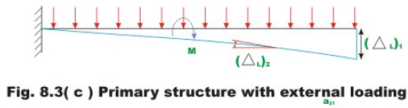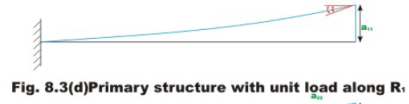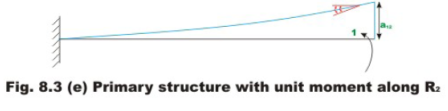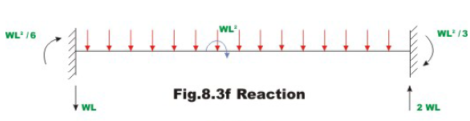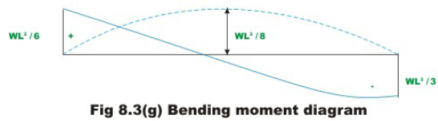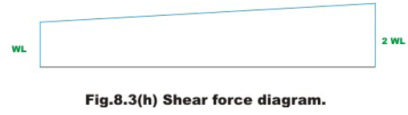The deflection (ΔL)1 and (ΔL)2 of the released structure can be evaluated from unit load method. Thus,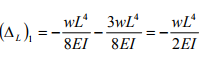(1)

and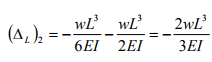(2)

The negative sign indicates that (ΔL)1 is downwards and rotation (ΔL)2 is clockwise. Hence the vector {ΔL} is given by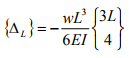(3)

The flexibility matrix is evaluated by first applying unit load along redundant R1 and determining the deflections a11 and a21 corresponding to redundants Rand R2 respectively (see Fig. 8.3d). Thus,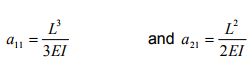(4)

Similarly, applying unit load in the direction of redundant R2,  one could evaluate flexibility coefficients a12 and a22 as as shown in Fig. 8.3c.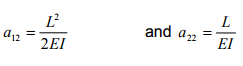(5)

Now the flexibility matrix is formulated as,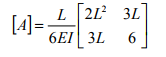(6)

The inverse of flexibility matrix is formulated as,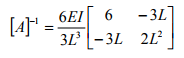The redundants are evaluated from equation (8.7). Hence,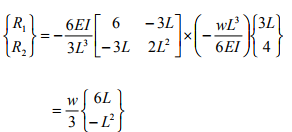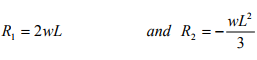(7)

The other two reactions (R3 and R4) can be evaluated by equations of statics. Thus,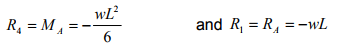(8)

The bending moment and shear force diagrams are shown in Fig. 8.3g and Fig.8.3h respectively.

Summary

In this lesson, statically indeterminate beams of degree more than one is solved systematically using flexibility matrix method. Towards this end matrix notation is adopted. Few illustrative examples are solved to illustrate the procedure. After analyzing the continuous beam, reactions are calculated and bending moment diagrams are drawn.

Offer running on EduRev: Apply code STAYHOME200 to get INR 200 off on our premium plan EduRev Infinity!

## Structural Analysis

30 videos|122 docs|28 tests

,

,

,

,

,

,

,

,

,

,

,

,

,

,

,

,

,

,

,

,

,

;